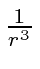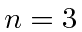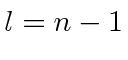## Homework Problems

1. Consider the fine structure of thestates of the hydrogen atom. What is the spectrum in the absence of a magnetic field? How is the spectrum changed when the atom is placed in a magnetic field of 25,000 gauss? Give numerical values for the energy shifts in each of the above cases. Now, try to estimate the binding energy for the lowest energystate including the relativistic, spin-orbit, and magnetic field.

2. Verify the relations used for,, andfor hydrogen atom states up toand for anyif.

3. Calculate the fine structure of hydrogen atoms for spin 1 electrons forand. Compute the energy shifts in eV.

Jim Branson 2013-04-22